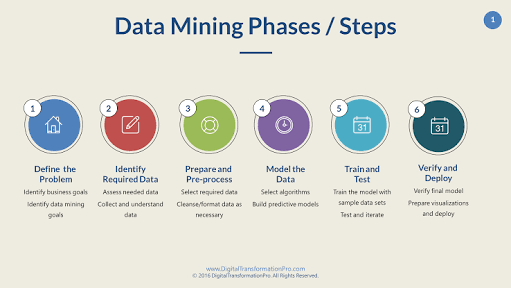# Intelligent Data Mining Assessment Test

10 Questions | Total Attempts: 244SettingsData mining is the process and practice of examining and sorting through large pre-existing data sets or databases in order to identify patterns and establish solutions to problems through data analysis, generate new information, and predict future trends.

• 1.
How is data stored in MOLAP?
• A.

Relational databases

• B.

Multidimensional databases

• C.

Single Dimensional databases

• D.

Distributed databases

• 2.
Which of the following is described as extraction of data from different sources?
• A.

Data merging

• B.

Data bagging

• C.

Data mining

• D.

Data dredging

• 3.
Deleting of data from data warehouse is called__
• A.

Data purging

• B.

Dara deletion

• C.

Data merging

• D.

Data booting

• 4.
Data cubes store data in___ version
• A.

Numerical

• B.

Compressed

• C.

Summarized

• D.

Alphabetical

• 5.
Which of the following is an example of discreet data?
• A.

Gender

• B.

Light

• C.

Height

• D.

Opinion

• 6.
Which of these help algorithm in pattern matching?
• A.

MODEL

• B.

Decision tree

• C.

MOLAP

• D.

OLAP

• 7.
Which of these can be used to predict continuous values of data?
• A.

Leaf

• B.

Decision tree

• C.

Time series

• D.

Node

• 8.
Naive Bayes Algorithm is used to
• A.

Change syntaxes

• B.

Create decision trees

• C.

Generate mining models

• D.

• 9.
Which of the following is used to group sets of data with similar characteristics?
• A.

Data merging

• B.

Cluster

• C.

Database

• D.

Decision tree

• 10.
Which of these algorithm is used for recommendation engine based on market analysis?
• A.

Sequence clustering algorithm

• B.

Association algorithm

• C.

Time series algorithm

• D.

Naive Bayes AlgorithmBack to top